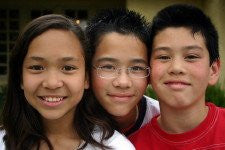## Discounted Upper Elementary Blackline Set

• 61600

• Fourth through sixth grade materials
• Third grade materials for reinforcement
• Seventh/eighth grade materials for enrichment
• Blackline masters for written work.
• Sets also include answer sheets.
• Numerous exercise sets

Thousands (green), Problem Solving C, Mixed Practice C, Geometry Introduction, Money C, Time C, Fraction Match, Roman Numerals, Problem Solving D, Mixed Practice D, Fraction Concepts, Decimal Introduction, Estimation, Advanced Numeration, Fraction Number Lines, Order Fractions, Fraction Operations, More Fraction Operations, Number Theory, Decimal Line & Labels, Order Decimals, Problem Solving E, Mixed Practice E, More Advanced Numeration, Decimal Fraction Equivalence, Percent, Geometry Intermediate, Advanced Decimals, Advanced Geometry, Intervals (Whole Numbers), Intervals (Positive Reals), Intervals (Integers), Intervals (All Reals), Operations with Negatives, Order of Operations, Order Reals, Algebra Concepts, Algebra Equations.